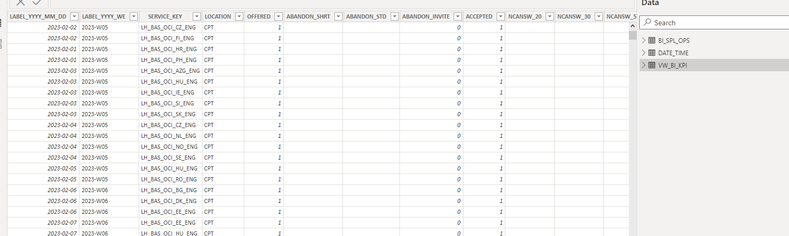cancel
Showing results for
Did you mean:New Member

## Same Y axis based on DAX MAX formula

Hi all,

I am trying to build a report which has multiple graphs showing accepted volume at the individual locations in our network.

Each graph is filtered down for a specific location and looks something like this:In order to show the correct proportion, I need the y-axis to be the same for all locations. Together with a colleague, we thought of using the MAX formula:

MAX = CALCULATE(MAX(VW_BI_KPI[ACCEPTED]),REMOVEFILTERS(VW_BI_KPI[LOCATION]))

which works fine, but...

Each location handles a multitude of different service keys. The above formula works out only if the data is filtered for one service key, but fails when it's two or more (it then gives the maximum accepted value for a single service key). I need two steps:

1) SUM of the volume per location across all service keys
2) the maximum of the above figure

The underlying data looks like this:Any suggestions how to achieve this?

Many thanks!

1 ACCEPTED SOLUTIONSuper User

Try

``````Max Y Axis =
VAR SummaryTable =
SUMMARIZE ( ALL ( VW_BI_KPI ), VW_BI_KPI[Location], 'Date'[Date] ),
"@value", CALCULATE ( SUM ( VW_BI_KPI[Accepted] ) )
)
VAR Result =
MAXX ( SummaryTable, [@value] )
RETURN
Result
``````
2 REPLIES 2Super User

Try

``````Max Y Axis =
VAR SummaryTable =
SUMMARIZE ( ALL ( VW_BI_KPI ), VW_BI_KPI[Location], 'Date'[Date] ),
"@value", CALCULATE ( SUM ( VW_BI_KPI[Accepted] ) )
)
VAR Result =
MAXX ( SummaryTable, [@value] )
RETURN
Result
``````New Member

Awesome, exactly what I needed! Thanks a lot!!Announcements#### Exclusive opportunity for Women!

Join us for a free, hands-on Microsoft workshop led by women trainers for women where you will learn how to build a Dashboard in a Day!#### Power Platform Conference-Power BI and Fabric Sessions

Join us Oct 1 - 6 in Las Vegas for the Microsoft Power Platform Conference.Top Solution Authors
Top Kudoed Authors
Users online (2,455)